AB Test Significance: Why mixing Bayesian & Frequentist is Best in A/B Testing

A/B testing allows marketers to harness the power of data in order to determine which version of a particular webpage is most successful in converting browsers into buyers.  But before conducting a complete website redesign, there are several crucial statistical decisions that must be made to correctly calculate and interpret the results: calculating the AB Test Significance is one of them.

It’s imperative to the success of your A/B Testing experiments that the data is properly applied to the appropriate statistical tests, and that the resulting information is then properly understood.

If results are interpreted incorrectly, you run the risk of implementing a website variation that has not mathematically proven itself to improve sales or leads. You even run the risk of decreasing conversions.

Even though crunching the numbers from your testing might seem like an objective task, there are actually a number of opinions as to how to go about collecting your results.

## AB Test Significance approaches: Frequentist or Bayesian

### The Frequentist Approach

The Frequentist approach is taken when only the raw data collected from an experiment is used to make predictions.

Say you want to see which webpage—A or B—performs best over the course of a particular Monday. At the end of that Monday, you take the data from the course of the day, calculate the AB Test Significance of a spike in conversions for page B, and determine that webpage B did indeed perform better than webpage A.

### The Bayesian Approach

The Bayesian approach is applied when previous data and results are considered alongside the raw data of the current experiment when drawing conclusions.

Applying this approach to the same experiment would mean considering traffic and conversion data from ten previous Mondays as well the current Monday in question.

## How can I choose which approach is right for me?

There are pros and cons to both approaches. The Frequentist, or “classical” approach, is faster and less complex, as it utilizes straightforward statistical calculations and a fixed data set that is only related to the specific experiment at hand. However, its limited data set poses more chances for the results to be merely due to chance.

The Bayesian approach is more complex, yet potentially more reliable. It relies on the assumption that if a certain outcome has been observed before, there’s a greater chance that it’ll happen again in the future.

For any philosophy buffs out there, Bayesian probability—coined in the 18th century by Presbyterian minister Thomas Bayes—is often believed to have been developed to counter David Hume’s argument that a “miraculous” event was unlikely to be a true miracle due to the innate rarity of a miracle in the first place.

Bayes potentially sought to challenge Hume by showing that future outcomes can be mathematically predicted by past occurrences, rather than an outcome’s perceived rarity.

As a 2014 New York Times article noted noted, even Hume himself might have been “impressed” when, in 2013, New York Coast Guard used Bayesian statistics to locate and rescue a fisherman who fell overboard in the Atlantic Ocean.

### So…which one should I use? Frequentist or Bayesian?

Well, A/B testing has given us the ability to statistically analyze more data points over a shorter period of time—the frequentist approach.

But it potentially prevents us from getting the most accurate results possible—if we “peek” at the results and see that AB Test Significance has been reached, we might truncate the experiment before it is truly over due to an error.

However, A/B testing also lets us introduce and accurately apply more complex statistical algorithms that take past outcomes into account—the Bayesian approach. But considering previous outcomes is more complicated and takes longer, and might be ignoring fresh data in favor of stale information.

The best approach to A/B testing statistical significance is, therefore, to use the best of both in what we at Convertize call the Hybrid Approach.

## What’s the ‘Hybrid approach’ and How Can it Help My Testing?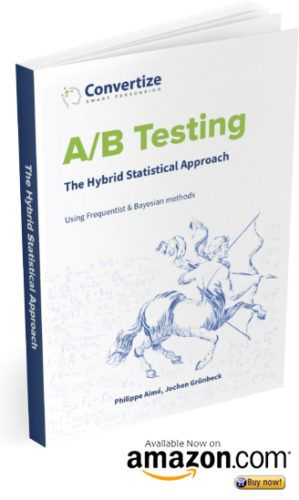Usually, statistical significance level in A/B testing is computed using a fixed sample size—attributed to the frequentist approach, since reducing the sample size can produce results faster.

This desire for quick results can lead to marketers calculating and checking the AB Test Significance result at the end of each running day of their experiment. Many statisticians advocate a “no peeking” rule, since marketers might be tempted to end an experiment the moment AB Test Significance is found, but before the targeted sample size has been reached.

This “no peeking” rule is due to a statistical phenomenon called “regression to the mean.”

This means that if you measure an extreme data point, the next measurement will be closer to the mean. Regression to the mean can happen to your measurements because of a sampling error, such as having sample that is too small, and therefore unrepresentative of the chosen population.

If you peek at the A/B results before the targeted sample size has been reached, and the results are unrepresentative of the true population due to regression to the mean, you’ll be lead to incorrect conclusions.

Since conversion rates, sample sizes, and therefore all the parameters in an A/B test continually evolve over the course of the experiment, we have decided to compute the significance level every running day only after a set number of days of testing in order to give our clients robust and reliable results.

We’ve programmed our algorithm to wait a specific number of running days before computing the significance level because of the volatility in data gathered at the beginning of the tests, due to regression to the mean and other errors. After these initial days, we calculate and provide the significance level at the end of every running day.

### Okay, I’m In — How can I Use The ‘Hybrid Approach’?

To successfully use the hybrid approach, it’s important to thoroughly understand the statistical tools used to calculate the results of A/B testing.

The following sections “A/B testing: The statistical basics” and “Applications in A/B testing” will go into detail regarding the basics of statistical significance, and will delve into the more complicated calculations in the section “How to calculate AB Test Significance in A/B tests using the hybrid approach.”

If you think you might need a refresher, read on…

## A/B Testing: The statistical basis & definitions

### What is AB Test Significance

In A/B testing, the most important tool for interpretation is Statistical Significance—the probability that the difference between the conversion rates of two webpage variations is the result of real changes in consumer behaviour. It’s a statistically robust way of proving that our results are reliable before jumping to conclusions.

Marketers and online web testers wait for a certain AB Test Significance level before choosing the winning variation. It is the easiest way of quantifying our level of certainty that we’ve received significant results once we analyze the data from our A/B test.

The AB Test Significance level most widely taken to indicate that there is a 95% chance that the results are significant. This means that 19 times out of 20, the variation that we have chosen as the winning one is the true winner. The probability that the results are irrelevant and merely due to chance is 1/20.

If your A/B test reveals that your results are statistically significant at a rate of p<0.05—probability of chance is less than 95%—then 19 times out of 20, when a visitor has completed a sale, it was on version “B” of your site rather than control version “A”.

Similarly, it means that 95% of the time, our results are not due to chance.

### What is the “Sample size”In statistics, the sample size is an important term that refers to the number of visitors used to collect data in our experiment. In the case of A/B testing, it means the number of people who have visited the two webpage variations.

In general, the larger the sample size, the more accurate the test will be.

The real danger with small datasets is that an “outlier”—or a data point that is very different in value from the rest of the results—will have a big impact on the interpreted results, and therefore the predictions.

### What is “The mean”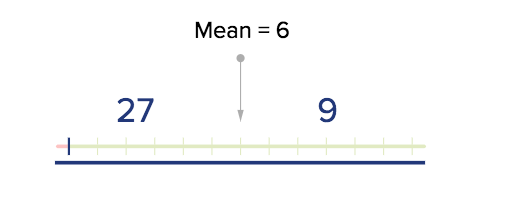The mean simply means “the average.” In A/B testing, we are measuring the mean conversion rate for each variation.

### What is the Variance & Standard Deviation

Variance – the difference between our results and expectation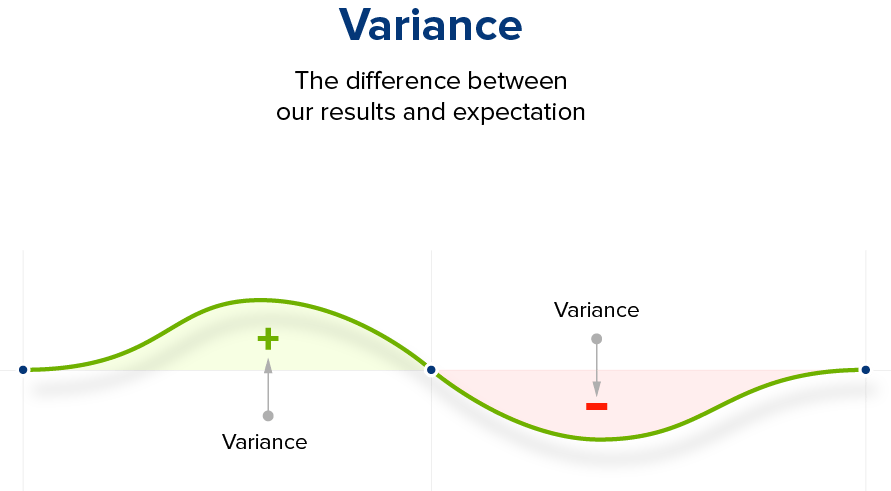The Variance measures the average spread between numbers in a dataset. It measures how far each number is from the mean. Usually, we aim to minimise the variance. The smaller the variance, the better the mean is a good guess of the typical conversion rate for a particular variation.

The standard deviation expresses how the data is clustered around the mean. The smaller the standard deviation, the closer our data set is to the mean.

Before we can prove that a hypothesis—an “educated guess”—is true, or even false, we need all of the data results to support this conclusion. We therefore will need to closely examine the population of our data set. In A/B testing our hypothesis is that the “B” page we optimize with nudge marketing notifications, calls-to-actions, customer reviews, etc. will perform better than the old page A.

Okay, got all of that? Great. It’s time to start testing.

### Testing our Hypothesis

In statistics, we use “hypothesis testing” to draw random samples from the population. This allows us to decide whether or not our hypothesis is true or false, and helps us avoid concluding results caused by change.

To do so, we first compare our educated guess—H1, the “alternative hypothesis”—to H0, the “null hypothesis.”

A null hypothesis states that there is no change in the data between the two populations in an experiment—e.g. webpages A and B in A/B testing showed the exact same conversion rates.

H1 is an alternative result to H0, which represents no change in the populations despite the experimental conditions.

H0= the null hypothesis
H1= the alternative hypothesis

What we want to test is if H1 is true.

In this case, 2 outcomes are possible:

1. We reject H0 and therefore accept H1 because we have sufficient supporting evidence.
2. We cannot reject H0 because not enough evidence.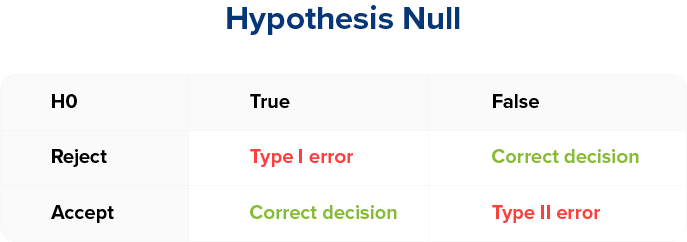The rejection of H0 even when H0 is true is called a Type I error.

Committing this error mean that in interpreting the results, we concluded that there was a change in conversion rates between webpages A and B during testing, even though the data reveals that there was not a change.

The probability of NOT committing this error is called the “statistical significance level” in marketers’ language.

In statistics, we define H0 in order to relate it to this kind error. The Type I error is usually the error that we most want to avoid because it is more important to not commit a Type I error than it is to commit a Type II error.

This is because committing a Type I error would mean seeing a change in one variation over another when there actually was no change in conversion rate between the two variation. Thus a marketer might accidentally implement changes perceived to increase sales when there is actually no evidence for this benefit.

The acceptance of H0 when H0 is false is the Type II error. The probability of NOT committing this error is called the “power.”

Relationship between type 1 and type 2 error rates: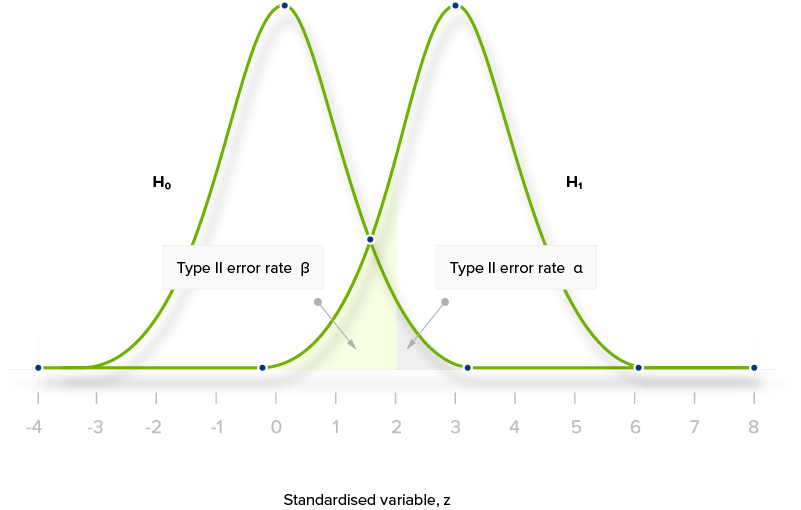It is important to notice that these two errors are antagonistic: we cannot try to reduce both Type I and Type II errors at the same time.

As you can see from the two bell curves, trying to decrease one of the two errors areas by moving “Any mean” will directly result in increasing the other type of error.

However, we can simultaneously reduce the probability of committing either of the two errors by increasing our sample size—in this case, the number of visitors to webpages A and B.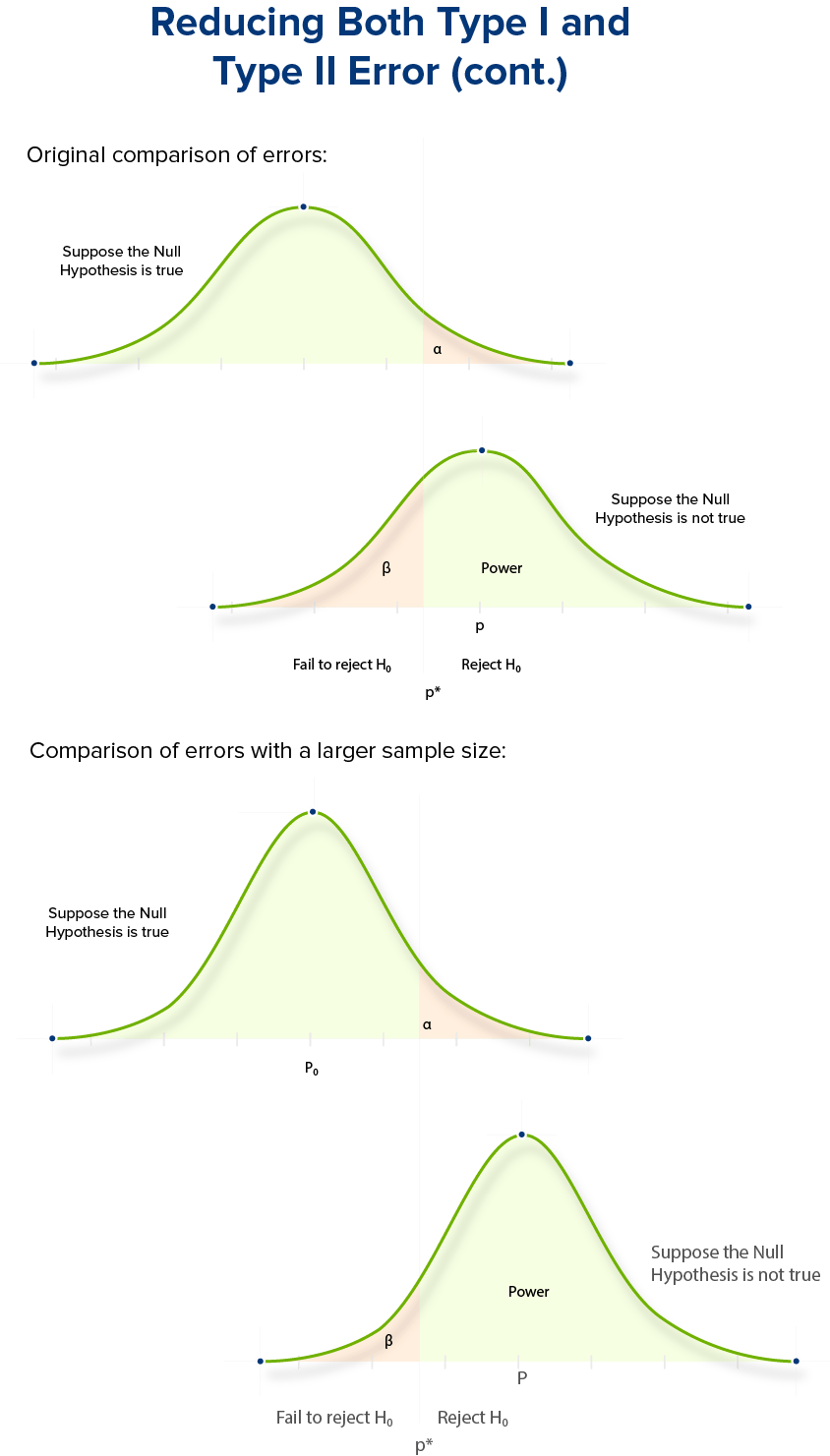## Going further with AB Test Significance and the Hybrid Approach